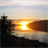# Subtract Two Rasters without Geoprocessing

57
0
10-15-2020 04:04 PMNew Contributor III

In Python you can simply subtract two rasters instead of using geoprocessing (raster calculator, subtract, minus)

``subtract_result = raster_one - raster_two‍‍``

Is there a way to do this with the ArcGIS Pro without using the SDK??

The SDK with geoprocessing being

``var valueArray = Geoprocessing.MakeValueArray(rasterOne, rasterTwo);var gpresult = await Geoprocessing.ExecuteToolAsync("Minus_sa", valueArray);‍‍‍‍``
0 Replies